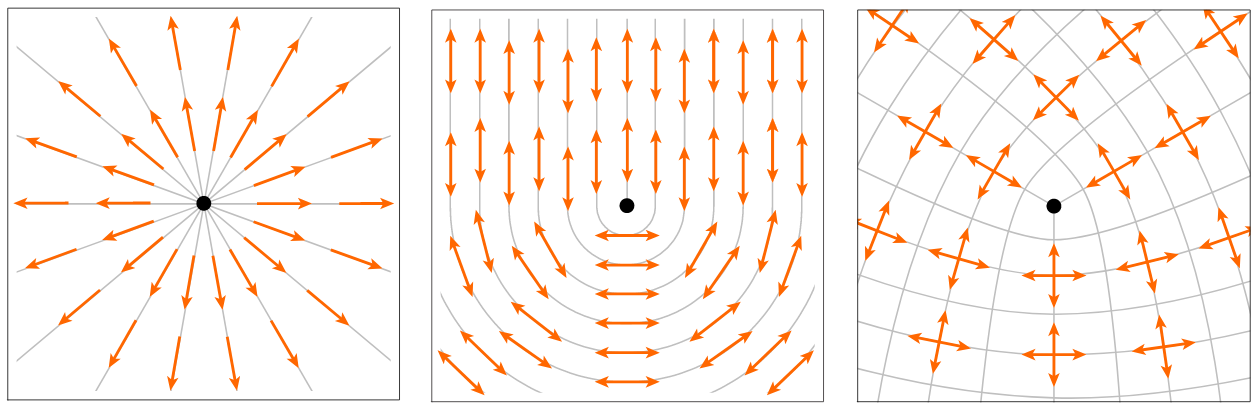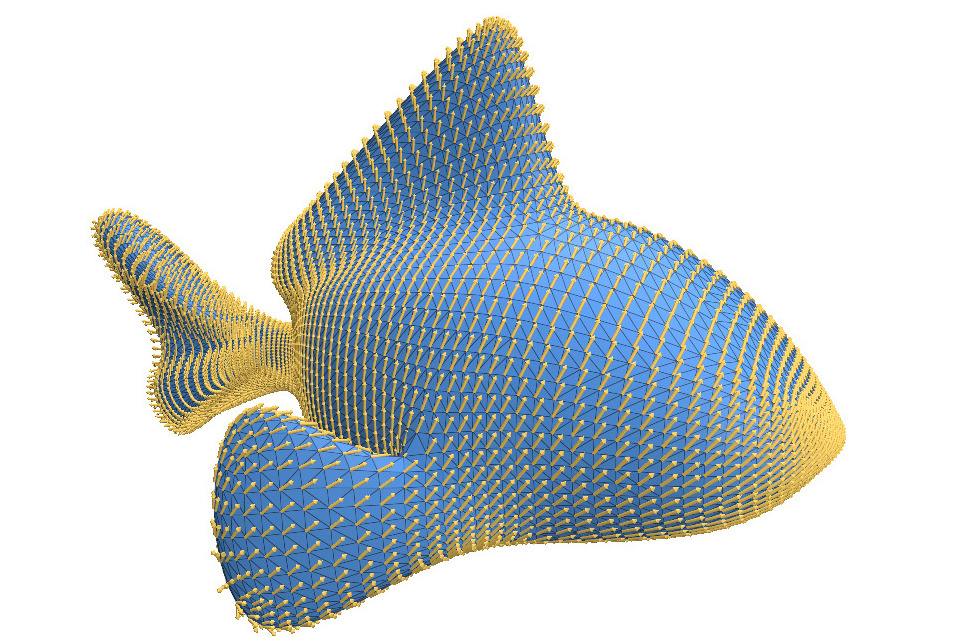# Direction Fields

This section describes routines for computing n-direction fields on a surface. An n-direction field on a surface assigns n evenly-spaced unit tangent vectors to each point on the surface. For example, a 1-direction field is an ordinary direction field, a 2-direction field is a line field, and a 4-direction field is a cross field.Most of these routines only depend on the intrinsic geometry of a surface (via the IntrinsicGeometryInterface). Therefore, you can run them on abstract geometric domains as well as traditional surfaces in 3D. However, a surface’s principal curvatures depend on the extrinsic geometry, so you can only compute curvature-aligned fields for surfaces in 3D.

#include "geometrycentral/surface/direction_fields.h"

How to interpret our symmetric direction fields
##### Interpreting symmetric direction fields

In geometry-central we use a “power” representation for symmetric vector fields (e.g. lines and cross fields, when n > 1 in the below algorithms).

For instance, in the case of cross fields n=4, there are four different tangent vectors at each point giving the resulting cross $v_0, v_1, v_2, v_3$. Rather than outputting any one of these vectors, we output a vector raised to the 4th power (where exponentiation is defined in the sense of complex numbers) $v = v_0^4 = v_1^4 = v_2^4 = v_3^4$. This representation makes sense, because after raising to the 4th power maps each of these four cross vectors to the same representative vector.

How do I get the cross/line/etc directions?

Concretely, to get out the four tangent direction vectors for a cross fields, one could do something like:

// Compute a cross field
int n = 4;
VertexData<Vector2> crossValues = computeSmoothestVertexDirectionField(*geometry, n);

for(Vertex v : mesh->vertices()) {

Vector2 representative = crossValues[v];
Vector2 crossDir = crossDir.pow(representative, 1. / n); // take the n'th root

// loop over the four directions
for(int rot = 0; rot < 4; rot++) {
// crossDir is one of the four cross directions, as a tangent vector
crossDir = crossDir.rot90();
}
}

(and the same applies when n = 2 for line fields, etc)


## Smoothest Direction FieldsThese routines compute the smoothest possible n-direction field on the input surface. They place singularities automatically. If you need to find out where the singularities are, refer to the Index Computation section below.

The two routines are almost identical. The only difference is that one discretizes direction fields using vectors at vertices, and the other uses vectors on faces.

Example

#include "geometrycentral/surface/direction_fields.h"
#include "geometrycentral/surface/meshio.h"
using namespace geometrycentral;
using namespace surface;

std::unique_ptr<ManifoldSurfaceMesh> mesh;
std::unique_ptr<VertexPositionGeometry> geometry;

// Compute a smooth direction field on vertices
VertexData<Vector2> directions = computeSmoothestVertexDirectionField(*geometry);
/* do something useful */


VertexData<Vector2> computeSmoothestVertexDirectionField(IntrinsicGeometryInterface& geometry, int nSym = 1)

Compute the smoothest n-direction field on the input surface.

FaceData<Vector2> computeSmoothestFaceDirectionField(IntrinsicGeometryInterface& geometry, int nSym = 1)

Compute the smoothest n-direction field on the input surface.

## Smoothest Boundary-Aligned Direction Fields

This routine works like the previous ones, except it imposes Dirichlet boundary conditions to force the generated direction field to be aligned with the mesh’s boundary. By default, the direction fields are aligned so that one of the field’s vectors is perpendicular to the boundary at each boundary vertex. If you set normalAlign to false then the direction fields are aligned so that one of the field’s vectors is parallel to the boundary instead.

VertexData<Vector2> computeSmoothestBoundaryAlignedVertexDirectionField(IntrinsicGeometryInterface& geometry, bool normalAlign = true, int nSym = 1)

Compute the smoothest n-direction field on the input surface, but ensures that the field is aligned with the surface’s boundary.

FaceData<Vector2> computeSmoothestBoundaryAlignedFaceDirectionField(IntrinsicGeometryInterface& geometry, int nSym = 1)

Compute the smoothest n-direction field on the input surface, but ensures that the field is aligned with the surface’s boundary.

## Curvature-Aligned Direction Fields

These routines compute smooth n-direction fields which align to the input surface’s principal curvatures. Since the principal curvatures form a 4-direction field, these methods only generate 2-direction fields and 4-direction fields.

Principal directions depend on the extrinsic geometry

Unlike the previous routines, computeCurvatureAlignedVertexDirectionField cannot operate using only the intrinsic geometry of its input surface. Since a surface’s principal directions depend on its embedding in 3D, these routines must take in an ExtrinsicGeometryInterface.

Curvature-alignment only works for 2- and 4-direction fields

VertexData<Vector2> computeCurvatureAlignedVertexDirectionField(ExtrinsicGeometryInterface& geometry, int nSym = 2)

Compute a smooth n-direction field on the input surface which is aligned to the surface’s principal curvatures. By default, n = 2.

FaceData<Vector2> computeCurvatureAlignedFaceDirectionField(ExtrinsicGeometryInterface& geometry, int nSym = 2)

Compute a smooth n-direction field on the input surface which is aligned to the surface’s principal curvatures. By default, n = 2.

## Index Computation

These methods compute the index of a given n-direction field at every point of the input mesh. If the direction field is represented by vector at vertices, then the singularities live on faces and vice versa.

FaceData<int> computeFaceIndex(IntrinsicGeometryInterface& geometry, const VertexData<Vector2>& directionField, int nSym = 1)

Compute the singularities in the input n-direction field. Since the direction field is given as vectors at vertices, the singularities are located on faces.

VertexData<int> computeVertexIndex(IntrinsicGeometryInterface& geometry, const FaceData<Vector2>& directionField, int nSym = 1)

Compute the singularities in the input n-direction field. Since the direction field is given as vectors at faces, the singularities are located on vertices.

## Citation

These algorithms are described in Globally Optimal Direction Fields, the appropriate citation is below. Note: these implementations do not currently exactly match what is described in the paper; they use a simpler “energy” via the vertex connection Laplacian.

@article{knoppel2013globally,
title={Globally optimal direction fields},
author={Kn{\"o}ppel, Felix and Crane, Keenan and Pinkall, Ulrich and Schr{\"o}der, Peter},
journal={ACM Transactions on Graphics (ToG)},
volume={32},
number={4},
pages={1--10},
year={2013},
publisher={ACM New York, NY, USA}
}